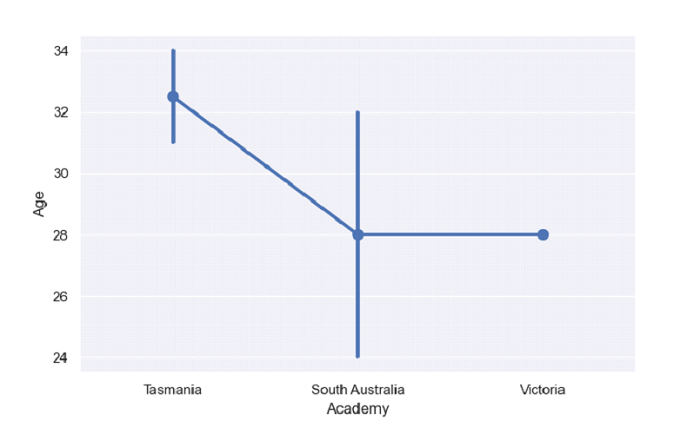# Python Pandas - Draw a point plot and control order by passing an explicit order with Seaborn

Point Plot in Seaborn is used to show point estimates and confidence intervals using scatter plot glyphs. The seaborn.pointplot() is used for this. For explicit order, use the order parameter of the pointplot() method.

Let’s say the following is our dataset in the form of a CSV file − Cricketers.csv

At first, import the required libraries −

import seaborn as sb
import pandas as pd
import matplotlib.pyplot as plt

Load data from a CSV file into a Pandas DataFrame −

dataFrame = pd.read_csv("C:\Users\amit_\Desktop\Cricketers.csv")

Plotting point plot with “Academy” and “Age”. Control order by passing an explicit order i.e. ordering on the basis of "Academy". Ordering using the order parameter −

sb.pointplot( x = 'Academy',y = 'Age', data = dataFrame, order=["Tasmania", "South Australia", "Victoria"] )

## Example

Following is the complete code −

import seaborn as sb
import pandas as pd
import matplotlib.pyplot as plt

# Load data from a CSV file into a Pandas DataFrame

sb.set_theme(style="darkgrid")

# plotting point plot with Academy and Age
# Control order by passing an explicit order i.e. ordering on the basis of "Academy"
# ordering using the order parameter
sb.pointplot( x = 'Academy',y = 'Age', data = dataFrame, order=["Tasmania", "South Australia", "Victoria"] )

# display
plt.show()

## Output

This will produce the following output −Updated on: 30-Sep-2021

229 Views